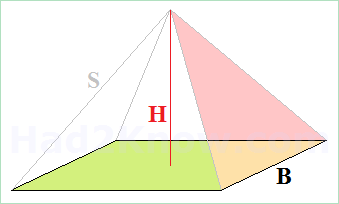# Square Pyramid Calculator

 Square Pyramid Calculator Base Length& Height B =  H = Base Length &Side Length B =  S = Height &Side Length H =  S =

Square pyramids were frequently used in ancient architecture and are one of the simplest shapes in solid geometry. The three main measures of a square pyramid are the base length B, height H, and triangular side length S. See figure below.

If you know any two of these measurements, you can compute the third. You can then compute the volume of the pyramid and the area of a triangular face once you know the values of B, H, and S. The equations are given below, or you may use the calculator at left.### Relations Among B, S, and H

For a square pyramid with a base length of B and a height of H, you can compute S with the formula

S = sqrt(B²/2 + H²).

If you know B and S and want to find H, the formula is

H = sqrt(S² - B²/2).

And finally, the formula for B in terms of H and S is

B = sqrt(2S² - 2H²).

### Area of a Triangular Face

Each face of a square pyramid is an isosceles triangle with sides S, S, and B. Using Heron's formula for triangular area, you can derive the expression

Area = (B)sqrt(S²/4 - B²/16).

### Volume of a Square Pyramid

The three-dimensional volume of a square pyramid is given by the equation

Volume = B²H/3.